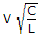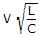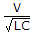# Electronics and Communication Engineering - Power Electronics

### Exercise :: Power Electronics - Section 6

36.

The total number of leads in MOS controlled thyristor is

 A. 2 B. 3 C. 4 D. 2 or 3

Explanation:

No answer description available for this question. Let us discuss.

37.

Assertion (A): Chopper always requires forced commutation

Reason (R): Morgan chopper uses a saturable reactor.

 A. Both A and R are correct and R is correct explanation of A B. Both A and R correct but R is not correct explanation of A C. A is correct but R is wrong D. A is wrong but R is correct

Explanation:

No answer description available for this question. Let us discuss.

38.

In a power diode the reverse recovery time is the time from the instant the forward current is zero to the instant when reverse recovery current has decayed to

 A. 50% of peak reverse current B. 25% of peak reverse current C. 10% of peak reverse current D. zero value

Explanation:

No answer description available for this question. Let us discuss.

39.

Assertion (A): McMurray Bredford half bridge inverter uses complementary commutation

Reason (R): Three phase inverter can have two modes of operation.

 A. Both A and R are correct and R is correct explanation of A B. Both A and R correct but R is not correct explanation of A C. A is correct but R is wrong D. A is wrong but R is correct

Explanation:

No answer description available for this question. Let us discuss.

40.

A capacitor C farads is charged to a voltage V. It discharges through a thyristor, a diode and an inductance L. The peak current in the circuit is

 A. VCL B.C.D.